# Lowest-Order Flows

To lowest order in the small parameter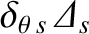, Equations (2.116) and (2.118) yield

 0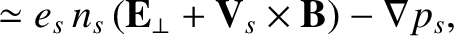(2.132) 0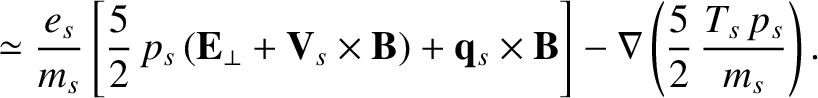(2.133)

The previous two equations can be solved to give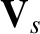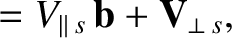(2.134)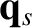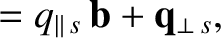(2.135)

where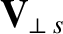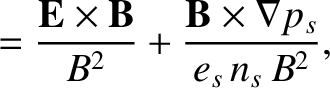(2.136)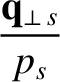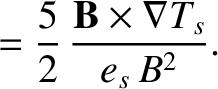(2.137)

It can be seen that the lowest-order particle and heat flows are both confined to magnetic flux-surfaces (i.e.,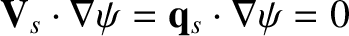). The first term on the right-hand side of Equation (2.136) is the E-cross-B velocity,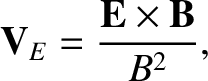(2.138)

that is common to all plasma species . The second term is the diamagnetic velocity,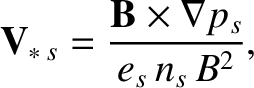(2.139)

which is different for electrons and ions, and is a consequence of the rapid gyro-motions of charged particles in the presence of equilibrium pressure gradients . The drift ordering (2.113) ensures that the E-cross-B and diamagnetic velocities are similar in magnitude. It is clear from Equation (2.137) that there is a diamagnetic flow of heat, as well as particles, around flux-surfaces; this heat flow is the same as that associated with the cross thermal conductivities introduced in Section 2.6.

To lowest order in the small parameter, Equations (2.115) and (2.117) yield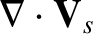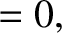(2.140)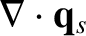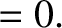(2.141)

Here, use has been made of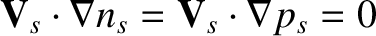. Clearly, the lowest-order particle and heat flows are both divergence free. Now, the fact thatimplies that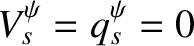. Making use of these results, the previous two equations can be combined with Equations (2.123) and (2.126), as well as the fact thatin an axisymmetric equilibrium, to give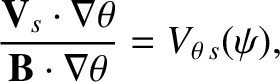(2.142)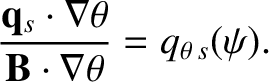(2.143)

Taking the scalar products of Equations (2.134) and (2.135) with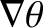, we obtain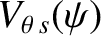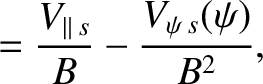(2.144)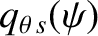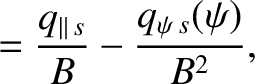(2.145)

where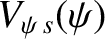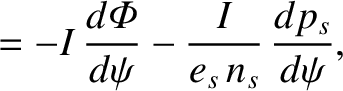(2.146)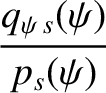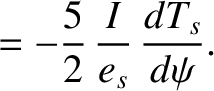(2.147)

Here, use has been made of Equations (2.136) and (2.137).